# Multi-Level Capacitor Level 0-3 w/Quantity (Version 8.0+)

The Multi-Level Capacitor has four model levels. As the level increases, additional parasitic circuit elements are added. This capacitor, available with Version 8.0 or later, is used to model a wide variety of capacitor types where the number and type of parasitic circuit elements can be configured.

In this topic:

 Model Name: Multi-Level Capacitor (Level 0-3 w/Quantity) Simulator: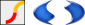This device is compatible with both the SIMetrix and SIMPLIS simulators. Parts Selector Menu Location (SIMetrix): Passives > Capacitors > Multi-Level Capacitor, US Style (V8+)Passives > Capacitors > Multi-Level Capacitor, EUR Style (V8+) Parts Selector Menu Location (SIMPLIS): Passives > Multi-Level Capacitor Level 0-3 w/Quantity (Version 8+) Symbol Library: passives.sxslb Model File (SIMetrix): preproc.lb Model File (SIMPLIS): simplis_param.lb Subcircuit Name: MULTI_LEVEL_CAP_QTY Symbol: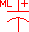Multiple Selections: Multiple devices can be selected and edited simultaneously.

## Editing the Multi-Level Capacitor

To configure the Multi-Level Capacitor, follow these steps:

1. Double click the symbol on the schematic to open the editing dialog to the Parameters tab.
2. Choose a model level, and then make the appropriate changes to the fields described in the table below the image.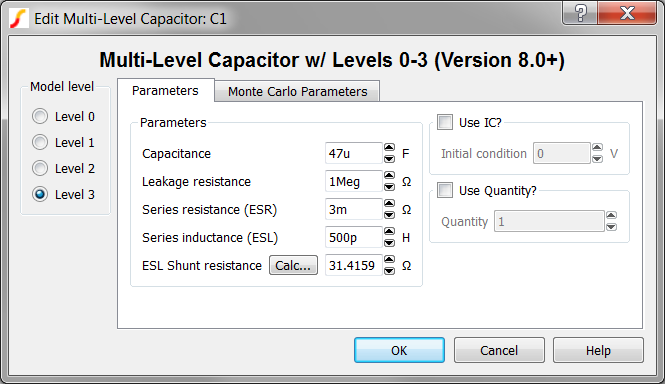Label Applicable Model Levels Units Description Capacitance 0,1,2,3 F Capacitance value Leakage resistance 1,2,3 Ω Leakage resistance across the capacitor Series resistance (ESR) 2,3 Ω The equivalent series resistance (ESR) Series inductance (ESL) 3 H The equivalent series inductance (ESL) ESL Shunt resistance 3 Ω The shunt resistance in parallel with the ESL. This resistance is included to limit the maximum frequency response of the parasitic ESL, which maximizes the simulation speed in SIMPLIS mode. Click Calc... to calculate this value. Use IC? all n/a If checked, the model uses the specified Initial condition. If unchecked, the capacitor initial condition is an open circuit. Initial condition all V Initial voltage of the capacitor at time=0 Use Quantity? all n/a If checked, the model uses the specified Quantity of capacitors in parallel. Quantity all n/a Number of capacitors in parallel. Note: To maximize simulation speed when using SIMPLIS, place a single symbol and specify a value for this parameter instead of placing multiple capacitors.

The model has built-in provisions for setting the Monte Carlo Parameters for all parameters except the ESL Shunt resistance. The Monte-Carlo Parameters are entered on the Monte Carlo Parameters tab shown below. To define the Monte Carlo Parameters follow these steps:

1. Click on the Monte Carlo Parameters tab.
2. Make the appropriate changes to the fields described in the table below the image.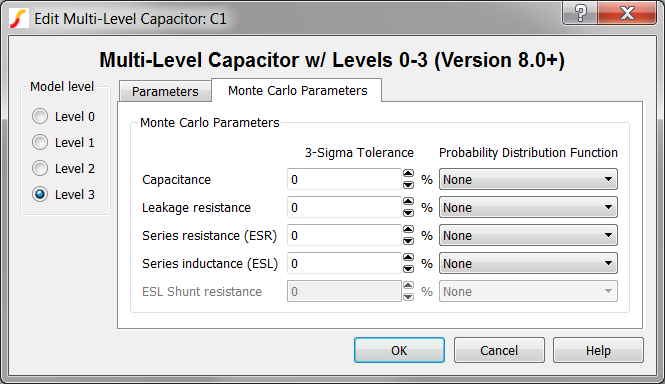Label Applicable Model Levels Units Description Capacitance 0,1,2,3 % 3-sigma tolerance for the capacitance value Leakage resistance 1,2,3 % 3-sigma tolerance for the leakage resistance across the capacitor Series resistance (ESR) 2,3 % 3-sigma tolerance for the equivalent series resistance (ESR) Series inductance (ESL) 3 % 3-sigma tolerance for the equivalent series inductance (ESL) Probability Distribution Function all n/a The choices for this function for each available parameter are the following: None The parameter has no tolerance and the value is fixed for Monte Carlo simulations. Gauss The parameter has a Gaussian distribution with the specified 3 sigma tolerance. Can return values greater than or less than the 3 sigma tolerance. Truncated Gauss As with Gauss but truncates all values outside the +/- 3 sigma tolerance. Uniform The parameter has a Uniform distribution within the specified 3 sigma tolerance. Worst Case The parameter has random values at the nominal value +/- the specified 3 sigma tolerance.

## Multi-Level Capacitor Model Levels

The Multi-Level capacitor has four levels: 0, 1, 2, and 3. As the model level increases, additional parasitic circuit elements are added to the model.

### Level 0 Model

Level 0 models an ideal capacitor with no parasitic elements.

Level 0 models these circuit elements Level 0 Schematic
 CAP Linear capacitance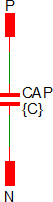### Level 1 Model

Level 1 models a capacitor with leakage resistance.

Level 1 models these circuit elements Level 1 Schematic
 CAP Linear capacitance RLK Leakage resistance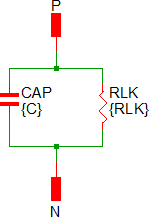### Level 2 Model

Level 2 models a capacitor with ESR and leakage resistance.

Level 2 models these circuit elements Level 2 Schematic
 CAP Linear capacitance RLK Leakage resistance RESR Equivalent series resistance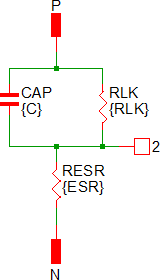### Level 3 Model

Level 3 models a capacitor with ESR, ESL, and leakage resistance.

Level 3 models these circuit elements Level 3 Schematic
 CAP Linear capacitance RLK Leakage resistance RESR Equivalent series resistance LESL Equivalent series inductance RESL ESL shunt resistance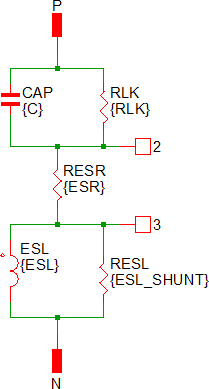## Quantity Implementation

### SIMPLIS Mode

The Multi-Level Capacitor model has two quantity parameters, USE_QTY and QTY, which specify the number of capacitors in parallel. Configuring these parameters minimizes the number of reactive circuit elements in the model and, therefore, provides a maximum simulation speed.

The implementation of the quantity parameter uses a "DC Transformer" technique where the capacitor's terminal current is multiplied by a constant using a Current Controlled Current Source (CCCS). This technique effectively divides all resistance and inductance values by the quantity parameter (QTY) and multiplies the capacitance by that same value. This circuitry is added if the USE_QTY parameter is set to 1.

A schematic of the quantity implementation for a Level 0 Multi-Level Capacitor is shown below: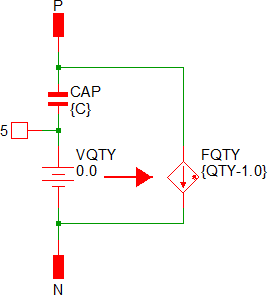### SIMetrix Mode

In SIMetrix, the quantity parameter is implemented with the "M" parameter which is common to the primitive SPICE components. This improves SIMetrix simulation speed and correctly scales resistances for noise analysis.

## Symbol Migration

Symbols placed on schematics in previous versions of SIMetrix/SIMPLIS can be automatically migrated to use the new symbols. The schematic tools menu Tools > Migrate Schematic Symbols to Version 8.00 will invoke a routine which migrates the existing symbols to the new symbols. As this action makes substantial changes to the schematic, it is recommended that you save a backup copy of the schematic first.